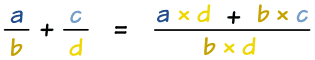# Using Rational Expressions

A Rational Expression is the ratio of two polynomials:## Using Rational Expressions

Using Rational Expressions is very similar to Using Rational Numbers (you may like to read that first).

The easiest way to add Rational Expressions is to use the common denominator method:Like in this example:

### Example:

2x−2 + 3x+1  =  2 × (x+1) + (x−2) × 3(x−2) × (x+1)

And then simplify to:

=   2x+2 + 3x−6 x2+x−2x−2

=   5x−4 x2−x−2

## Subtracting Rational Expressions

### Example:

2 x−2 3 x+1   =   2 × (x+1) − (x−2) × 3 (x−2) × (x+1)

And then simplify to:

=   2x+2 − (3x−6) x2+x−2x−2

=   −x + 8 x2−x−2

## Multiplication

To multiply two Rational Expressions, just multiply the tops and bottoms separately, like this:

### Example:

2x−2  ×  3x+1  =  2×3(x−2)(x+1)

And then simplify to:

=  6x2−x−2

## Division

To divide two Rational Expressions, first flip the second expression over (make it a reciprocal) and then do a multiply like above:

### Example:

First flip the second one over and make it a multiply:

2x2   /   3x+1  =  2x2  ×  x+13

Then do the multiply:

2 x2   ×  x+13  =  2(x+1)3(x2)

## Simplifying

When simplifying a rational function be careful to respect where the lower polynomial is equal to zero

### Example:

x2−1x+1 is undefined when x=−1

Its Domain (the values that can go into the expression) does not include −1

Now, we can factor x2−1 into (x−1)(x+1) so we get:

(x−1)(x+1)(x+1)

It is now tempting to cancel (x+1) from top and bottom to produce:

x − 1

Its Domain now does include −1

But it is now a different function because it has a different Domain.